Documentation

### This is machine translation

Mouseover text to see original. Click the button below to return to the English version of the page.

To view all translated materials including this page, select Country from the country navigator on the bottom of this page.

## Structural Dynamics of Tuning Fork

Perform modal and transient analysis of a tuning fork.

A tuning fork is a U-shaped beam. When struck on one of its prongs or tines, it vibrates at its fundamental (first) frequency and produces an audible sound.

The first flexible mode of a tuning fork is characterized by symmetric vibration of the tines: they move towards and away from each other simultaneously, balancing the forces at the base where they intersect. The fundamental mode of vibration does not produce any bending effect on the handle attached at the intersection of tines. The lack of bending at the base enables easy handling of tuning fork without influencing its dynamics.

Transverse vibration of the tines causes the handle to vibrate axially at the fundamental frequency. This axial vibration can be used to amplify the audible sound by bringing the end of the handle in contact with a larger surface area, like a metal table top. The next higher mode with symmetric mode shape is about 6.25 times the fundamental frequency. Therefore, a properly excited tuning fork tends to vibrate with a dominant frequency corresponding to fundamental frequency, producing a pure audible tone. This example simulates these aspects of the tuning fork dynamics by performing a modal analysis and a transient dynamics simulation.

You can find the helper functions `animateSixTuningForkModes` and `tuningForkFFT` and the geometry file `TuningFork.stl` under `matlab/R20XXx/examples/pde/main`.

### Modal Analysis of Tuning Fork

Find natural frequencies and mode shapes for the fundamental mode of a tuning fork and the next several modes. Show the lack of bending effect on the fork handle at the fundamental frequency.

First, create a structural model for modal analysis of a solid tuning fork.

`model = createpde('structural','modal-solid');`

To perform unconstrained modal analysis of a structure, it is enough to specify geometry, mesh, and material properties. First, import and plot the tuning fork geometry.

```importGeometry(model,'TuningFork.stl'); figure pdegplot(model)```Specify the Young's modulus, Poisson's ratio, and mass density to model linear elastic material behavior. Specify all physical properties in consistent units.

```E = 210E9; nu = 0.3; rho = 8000; structuralProperties(model,'YoungsModulus',E, ... 'PoissonsRatio',nu, ... 'MassDensity',rho);```

Generate a mesh.

`generateMesh(model,'Hmax',0.001);`

Solve the model for a chosen frequency range. Specify the lower frequency limit below zero so that all modes with frequencies near zero appear in the solution.

`RF = solve(model,'FrequencyRange',[-1,4000]*2*pi);`

By default, the solver returns circular frequencies.

`modeID = 1:numel(RF.NaturalFrequencies);`

Express the resulting frequencies in Hz by dividing them by. Display the frequencies in a table.

```tmodalResults = table(modeID.',RF.NaturalFrequencies/2/pi); tmodalResults.Properties.VariableNames = {'Mode','Frequency'}; disp(tmodalResults);```
``` Mode Frequency ____ _________ 1 0.0011196 2 0.0032769 3 0.0044698 4 0.0077204 5 0.0092536 6 0.012323 7 460.42 8 706.34 9 1911.5 10 2105.5 11 2906.5 12 3814.7 ```

Because there are no boundary constraints in this example, modal results include the rigid body modes. The first six near-zero frequencies indicate the six rigid body modes of a 3-D solid body. The first flexible mode is the seventh mode with a frequency around 460 Hz.

The best way to visualize mode shapes is to animate the harmonic motion at their respective frequencies. The `animateSixTuningForkModes` function animates the six flexible modes, which are modes 7 through 12 in the modal results `RF`.

`frames = animateSixTuningForkModes(RF);`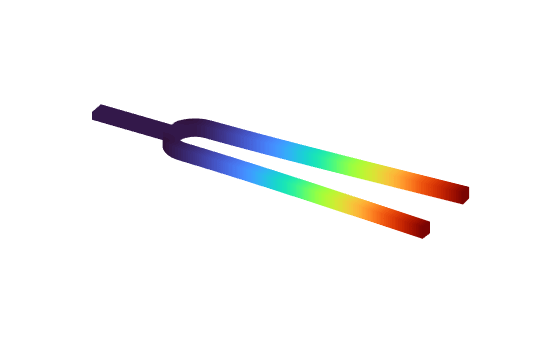To play the animation, use the following command:

`movie(figure('units','normalized','outerposition',[0 0 1 1]),frames,5,30)`

In the first mode, two oscillating tines of the tuning fork balance out transverse forces at the handle. The next mode with this effect is the fifth flexible mode with the frequency 2906.5 Hz. This frequency is about 6.25 times greater than the fundamental frequency 460 Hz.

### Transient Analysis of Tuning Fork

Simulate the dynamics of a tuning fork being gently and quickly struck on one of its tines. Analyze vibration of tines over time and axial vibration of the handle.

First, create a structural transient analysis model.

`tmodel = createpde('structural','transient-solid');`

Import the same tuning fork geometry you used for the modal analysis.

`importGeometry(tmodel,'TuningFork.stl');`

Generate a mesh.

`mesh = generateMesh(tmodel,'Hmax',0.005);`

Specify the Young's modulus, Poisson's ratio, and mass density.

```structuralProperties(tmodel,'YoungsModulus',E, ... 'PoissonsRatio',nu, ... 'MassDensity',rho);```

Identify faces for applying boundary constraints and loads by plotting the geometry with the face labels.

```figure('units','normalized','outerposition',[0 0 1 1]) pdegplot(tmodel,'FaceLabels','on') view(-50,15) title 'Geometry with Face Labels'```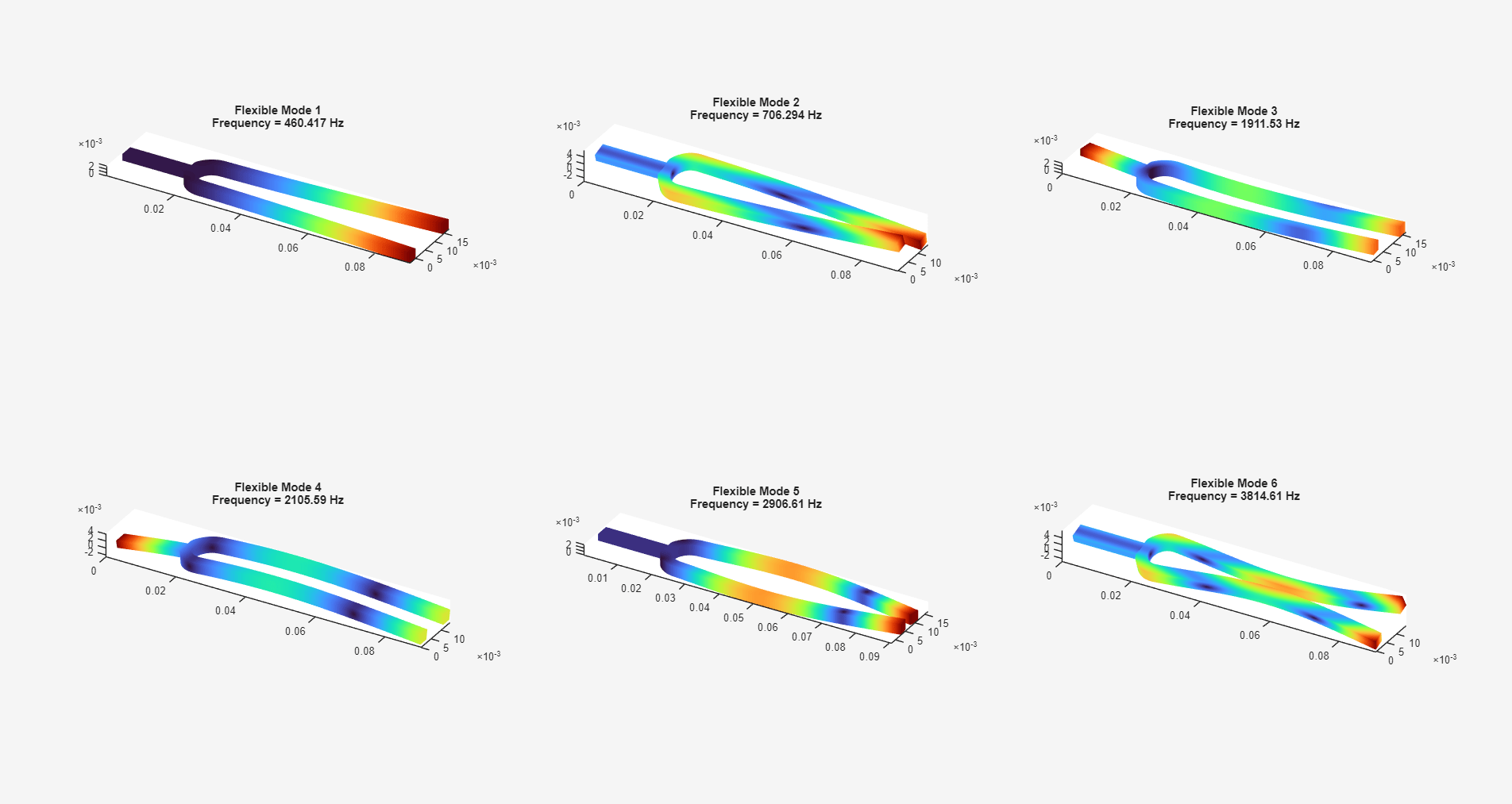Impose sufficient boundary constraints to prevent rigid body motion under applied loading. Typically, you hold a tuning fork by hand or mount it on a table. A simplified approximation to this boundary condition is fixing a region near the intersection of tines and the handle (faces 21 and 22).

`structuralBC(tmodel,'Face',[21,22],'Constraint','fixed');`

Approximate an impulse loading on a face a tine by applying a pressure load for a very small fraction of the time period of the fundamental mode. By using this very short pressure pulse, you ensure that only the fundamental mode of a tuning fork is excited. To evaluate the time period `T` of the fundamental mode, use the results of modal analysis.

`T = 2*pi/RF.NaturalFrequencies(7);`

Specify the pressure loading on a tine as a short rectangular pressure pulse.

`structuralBoundaryLoad(tmodel,'Face',11,'Pressure',5E6,'EndTime',T/300);`

Apply zero displacement and velocity as initial conditions.

`structuralIC(tmodel,'Displacement',[0;0;0],'Velocity',[0;0;0]);`

Solve the transient model for 50 periods of the fundamental mode. Sample the dynamics 60 times per period of the fundamental mode.

```ncycle = 50; sampingFrequency = 60/T; tlist = linspace(0,ncycle*T,ncycle*T*sampingFrequency); R = solve(tmodel,tlist)```
```R = TransientStructuralResults with properties: Displacement: [1x1 struct] Velocity: [1x1 struct] Acceleration: [1x1 struct] SolutionTimes: [1x2999 double] Mesh: [1x1 FEMesh] ```

Plot the time-series of the vibration of the tine tip, which is face 12. Find nodes on the tip face and plot the `y`-component of the displacement over time, using one of these nodes.

```excitedTineTipNodes = findNodes(mesh,'region','Face',12); tipDisp = R.Displacement.uy(excitedTineTipNodes(1),:); figure plot(R.SolutionTimes,tipDisp) title('Transverse Displacement at Tine Tip') xlim([0,0.1]) xlabel('Time') ylabel('Y-Displacement')```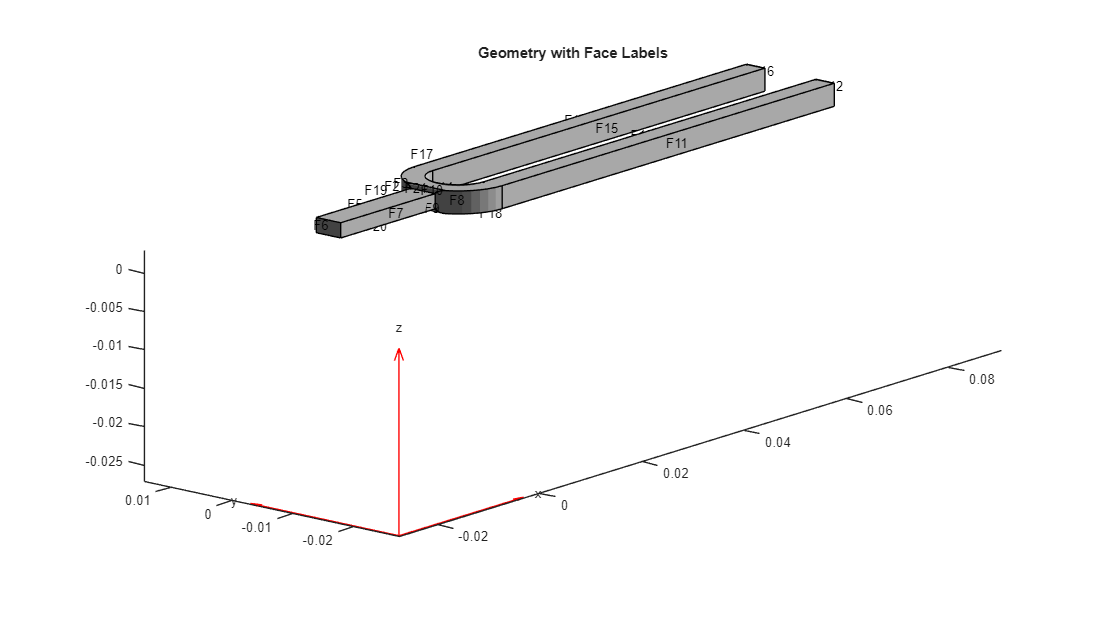Perform fast Fourier transform (FFT) on the tip displacement time-series to see that the vibration frequency of the tuning fork is close to its fundamental frequency. A small deviation from the fundamental frequency computed in an unconstrained modal analysis appears because of constraints imposed in the transient analysis.

```[fTip,PTip] = tuningForkFFT(tipDisp,sampingFrequency); figure plot(fTip,PTip) title({'Single-sided Amplitude Spectrum', 'of Tip Vibration'}) xlabel('f (Hz)') ylabel('|P1(f)|') xlim([0,4000])```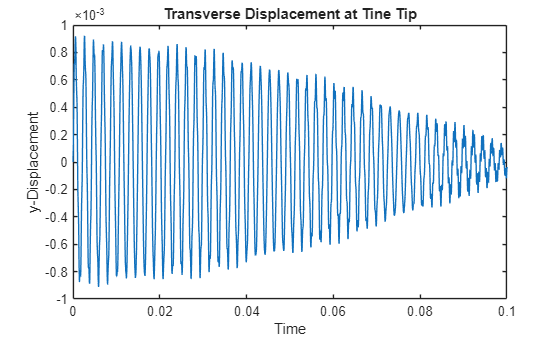Transverse vibration of tines causes the handle to vibrate axially with the same frequency. To observe this vibration, plot the axial displacement time-series of the end face of the handle.

```baseNodes = tmodel.Mesh.findNodes('region','Face',6); baseDisp = R.Displacement.ux(baseNodes(1),:); figure plot(R.SolutionTimes,baseDisp) title('Axial Displacement at the End of Handle') xlim([0,0.1]) ylabel('X-Displacement') xlabel('Time')```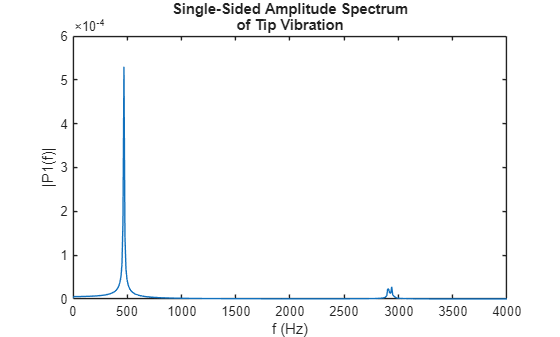Perform an FFT of the time-series of the axial vibration of the handle. This vibration frequency is also close to its fundamental frequency.

```[fBase,PBase] = tuningForkFFT(baseDisp,sampingFrequency); figure plot(fBase,PBase) title({'Single-sided Amplitude Spectrum', 'of Base Vibration'}) xlabel('f (Hz)') ylabel('|P1(f)|') xlim([0,4000]) ```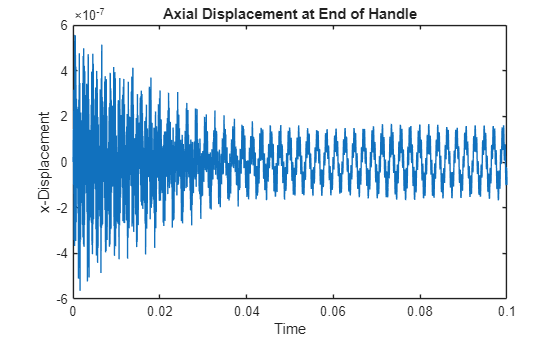##### SupportGet trial now